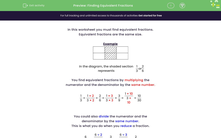# Find Equivalent Fractions

In this worksheet, students will practise finding equivalent fractions.Key stage:  KS 3

Curriculum topic:   Ratio, Proportion and Rates of Change

Curriculum subtopic:   Relate Ratio Language to Fractions and Linear Functions

Difficulty level:#### Worksheet Overview

In this activity, we will be finding equivalent fractions.

Equivalent fractions are the same size.

Example

In the diagram below, the shaded section represents 2/6 or 1/3You find equivalent fractions by multiplying the numerator and the denominator by the same number.

 1 3
=
 1 × 2 3 × 2
=
 2 6
=
 1 × 3 3 × 3
=
 3 9
=
 1 × 10 3 × 10
=
 10 30

You can also divide the numerator and the denominator by the same number.

This is what you do when you reduce or simplify a fraction.

 6 24
=
 6 ÷ 2 24 ÷ 2
=
 3 12
=
 6 ÷ 3 24 ÷ 3
=
 2 8

Let's try some questions now.

### What is EdPlace?

We're your National Curriculum aligned online education content provider helping each child succeed in English, maths and science from year 1 to GCSE. With an EdPlace account you’ll be able to track and measure progress, helping each child achieve their best. We build confidence and attainment by personalising each child’s learning at a level that suits them.

Get started